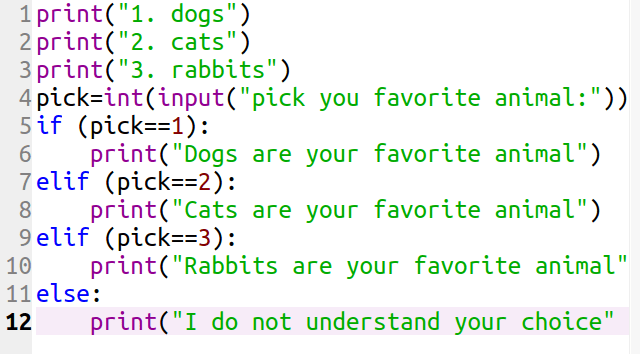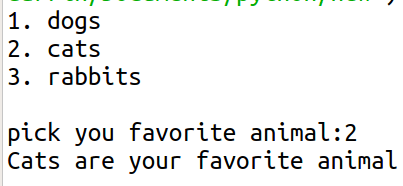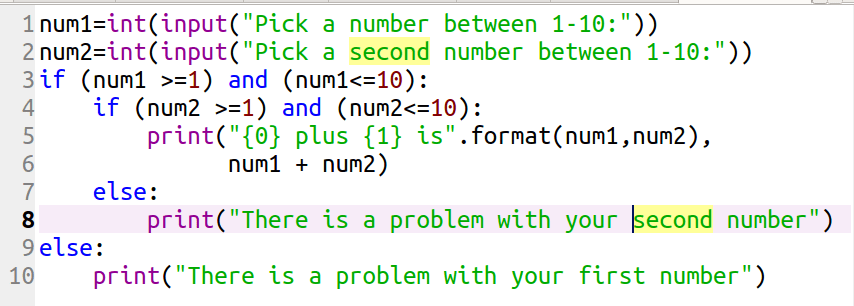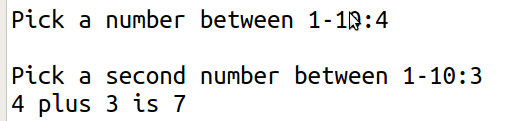# elif Clause and Nested Statements in Python

This post will provide a brief introduction into the use of the elif clause and nested statements in Python.

elif ClauseThe elif clause is used to add an additional set of conditions to an if statement. If you have ever used some sort of menu on a computer in which you had to make several choices it is possible that an elif clause was involved in the code.

The syntax for the elif clause is the same as for the if statement. Below is a simple example that employs the elif clause.

``

Here is what this code does.

1. In lines 1-3, I print three lines of code at the beginning. These are the choices available to the user.
2. In line 4, the “pick” variable stores whatever number the user inputs through the “input” function. It must be an integer which is why I used the “int” function
3. In line 5 we begin the if statement. If the “pick” variable is set to 1 you can see the printout in line 6.
4. In lines 7 and 8 we use the elif clause. The settings are mostly the same as in the if statement in line 5 except the “pick” variable is set to different numbers with different outputs.
5. Lastly, in line 11 we have the else clause. If for any reason the person picks something besides 1,2 or 3 this message will appear.

What this code means in simple English is this

• If the pick is 1 then print “dogs are your favorite animal”
• or else if the pick is 2 then print “cats are your favorite animal”
• or else if the pick is 3 then print “rabbits are your favorite animal”
• else print “I do not understand your choice”

Here is what the code looks like when you run itAs a note, if you type in a letter or string you will get an error message. This is because our code is not sophisticated enough to deal with non-integers at this point.

Nested Statements

Just like with functions which can be nested so can decision statements by nesting inside each other. To put this simply the conditions set by the first if statement can potentially affect the second condition. This is explained better with an example.Here is what is happening in the code above.

1. In lines 1 and 2 the user has to pick numbers that are saved in the variables “num1” and “num2.”
2. Line 3 and 4 are the if statements. Line 3 and line 9 are the outer if statement and line 4-8 are the inner if statement.
3. Line 3 shares that “num1” must be between 1 and 10.
4. Line 4 shares that “num2” must be between 1 and 10.
5. Line 5 is the results of the inner if statement. The results are printed using the “.format” method where the {0} and {1} are the variables “num1” and “num2”. After the comma is what is done to the variables, they are simply added together.
6. Line 8 is the else clause. If someone types something different form a number between 1-10 for the second number they will see this message
7. Line 9 is the else clause for the outer if statement. This is only seen if a value different from 1-10 is inputted.

If you run the code here is what it should look likeConclusion

The elif clause and nested decision statements are additional tools that can be used to empower your applications. This is some of the most basic ideas in using a language such as Python.